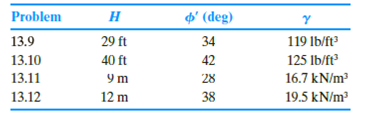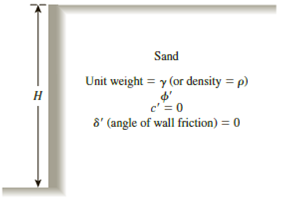Chapter 13, Problem 13.12PPrinciples of Geotechnical Enginee...

9th Edition
Braja M. Das + 1 other
ISBN: 9781305970939

Solutions

Chapter
SectionPrinciples of Geotechnical Enginee...

9th Edition
Braja M. Das + 1 other
ISBN: 9781305970939
Textbook Problem

13.9 through 13.12 Assume that the retaining wall shown in Figure 13.39 is frictionless. For each problem, determine the Rankine active force per unit length of the wall, the variation of active earth pressure with depth, and the location of the resultant.Figure 13.39

To determine

The Rankine active force σa(z=H) per unit length of the wall, the variation of active earth pressure Pa with depth, and the location z¯ of the resultant measured from the bottom of the wall.

Explanation

Given information:

The height (H) of the retaining wall is 12.0 m.

The soil friction angle ϕ is 38°.

The angle of wall friction δ is 0.

The unit weight γ of the soil is 19.5kN/m3

Calculation:

Calculate the active earth pressure coefficient (Ka) using the relation.

Ka=tan2(45ϕ2)

Substitute 38° for ϕ.

Ka=tan2(4538°2)=tan2(4519)=tan2(26)=0.238

Calculate Rankine active force σa(z=H) per unit length of the wall using the relation.

σa(z=H)=KaγH

Substitute 0.238 for Ka, 19.5lb/ft3 for γ, and 12 m for H.

σa(z=H)=0

Still sussing out bartleby?

Check out a sample textbook solution.

See a sample solution

The Solution to Your Study Problems

Bartleby provides explanations to thousands of textbook problems written by our experts, many with advanced degrees!

Get Started

Find more solutions based on key concepts

Database Systems: Design, Implementation, & Management

Which device may be used for either toolholding or workholding?

Precision Machining Technology (MindTap Course List)

Why would a manager prefer a decision tree instead of a decision table?

Systems Analysis and Design (Shelly Cashman Series) (MindTap Course List)

Describe an ideal tack weld.

Welding: Principles and Applications (MindTap Course List)

How do contactless smart cards communicate with a reader? (393)

Enhanced Discovering Computers 2017 (Shelly Cashman Series) (MindTap Course List)